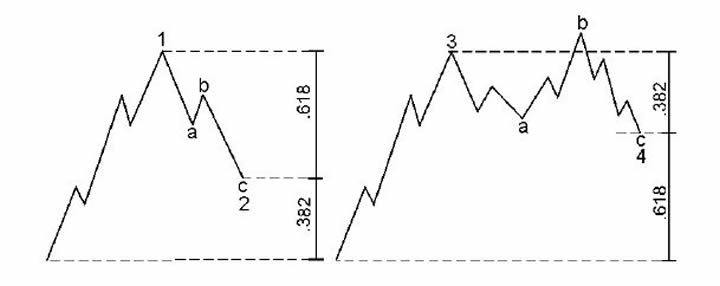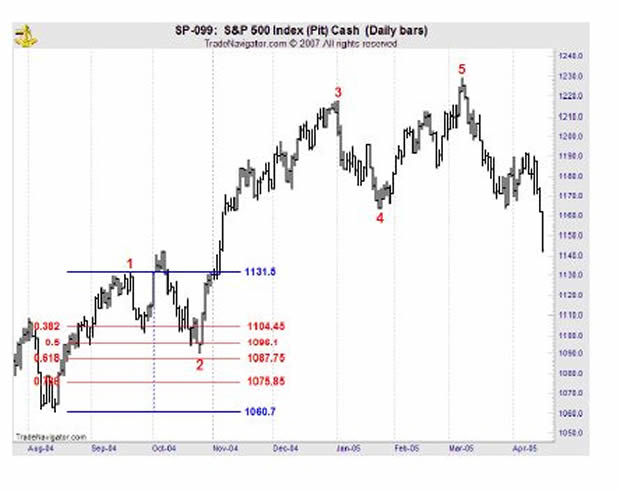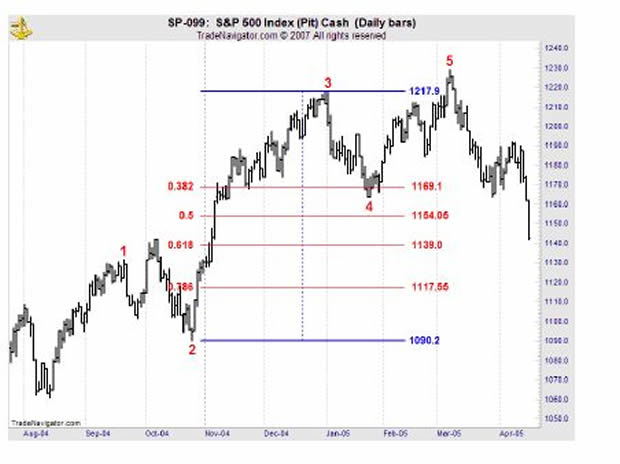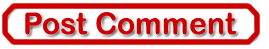Best of the Week# Learn How to Apply Fibonacci Retracements to Your Stock Index Trading

InvestorEducation / Learn to Trade Jun 19, 2015 - 11:12 PM GMT

By: EWIFibonacci is the mathematical basis of the Wave Principle. You will often find that Elliott waves correct in terms of Fibonacci ratios. The following article explains what you can expect when a market begins a corrective phase.

Retracements -- Corrective WavesThe chart on the left shows a wave 1 followed by corrective wave 2. It is common for second waves to retrace .618 of wave 1 -- thereby making a deep retracement. Another common retracement for wave 2 is .786. You might even see .5, 50%, but .618 is more common. The chart on the right shows the most common retracement for a wave 4. Fourth waves will commonly retrace a smaller percentage or .382 of wave 3. We might also see something like .236.

Examples

Below you can see an impulsive 5-wave move on the chart of the S&P 500. Wave 2 is an expanded flat. Wave 4 is a zigzag. Let's look at the retracements that waves 2 and 4 make.Wave 2 made a deep retracement -- close to .618. On the Fibonacci table you can see the .382, .5, .618, and .786 retracements. The .618 retracement comes in at 1087.75 and the S&P low is 1090.19.Wave 4 made a shallow retracement of wave 3. It went just beyond the .382 retracement at 1169.1, bottoming at 1163.75.

In a nutshell, this is what we mean when we say that Elliott waves often correct in terms of Fibonacci ratios.EWI Senior Tutorial Instructor Wayne Gorman explains:

• The Golden Spiral, the Golden Ratio, and the Golden Section
• How to use Fibonacci Ratios/Multiples in forecasting
• How to identify market targets and turning points in the markets you trade
• And more!